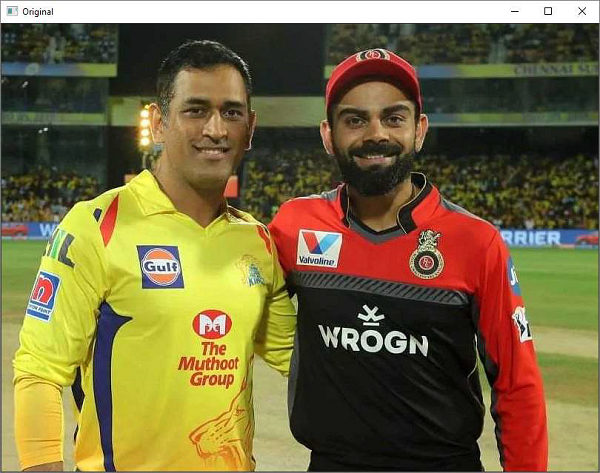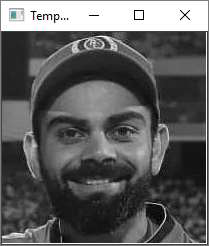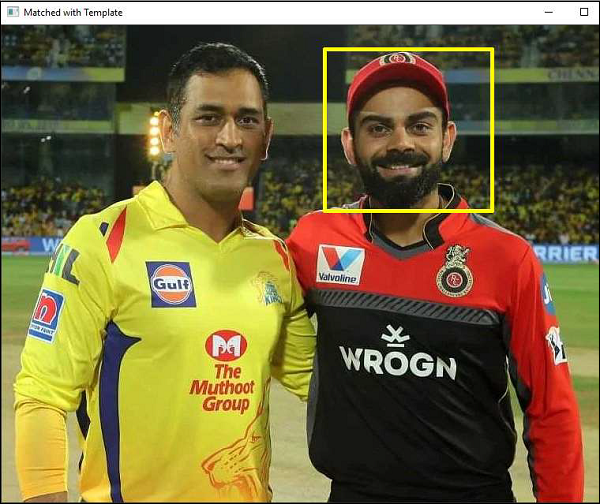# OpenCV Python - Template Matching

The technique of template matching is used to detect one or more areas in an image that matches with a sample or template image.

Cv.matchTemplate() function in OpenCV is defined for the purpose and the command for the same is as follows:

cv.matchTemplate(image, templ, method)


Where image is the input image in which the templ (template) pattern is to be located. The method parameter takes one of the following values −

• cv.TM_CCOEFF,
• cv.TM_CCOEFF_NORMED, cv.TM_CCORR,
• cv.TM_CCORR_NORMED,
• cv.TM_SQDIFF,
• cv.TM_SQDIFF_NORMED

This method slides the template image over the input image. This is a similar process to convolution and compares the template and patch of input image under the template image.

It returns a grayscale image, whose each pixel denotes how much it matches with the template. If the input image is of size (WxH) and template image is of size (wxh), the output image will have a size of (W-w+1, H-h+1). Hence, that rectangle is your region of template.

## Example

In an example below, an image having Indian cricketer Virat Kohli’s face is used as a template to be matched with another image which depicts his photograph with another Indian cricketer M.S.Dhoni.

Following program uses a threshold value of 80% and draws a rectangle around the matching face −

import cv2
import numpy as np

cv2.imshow('Original',img)
gray = cv2.cvtColor(img, cv2.COLOR_BGR2GRAY)

cv2.imshow('Template',template)
w,h = template.shape, template.shape

matched = cv2.matchTemplate(gray,template,cv2.TM_CCOEFF_NORMED)
threshold = 0.8

loc = np.where( matched >= threshold)

for pt in zip(*loc[::-1]):
cv2.rectangle(img, pt, (pt + w, pt + h), (0,255,255), 2)

cv2.imshow('Matched with Template',img)


## Output

The original image, the template and matched image of the result as follows −

### Original imageThe template is as follows −The image when matched with template is as follows −